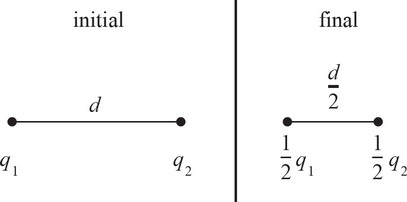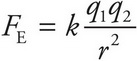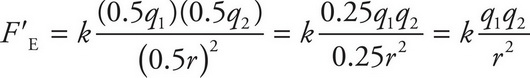# AP Physics 1 Question 458: Answer and Explanation

### Test Information

Question: 4586. What happens to the magnitude of the electric force between two opposite charges if the magnitude of each charge is halved and the distance between their centers is halved?

• A. It is doubled.
• C. It is halved.
• D. It remains the same.

Explanation:

D

The electric force between two particles with charges of q1 and q2, separated by a distance r, is given by the following equation:If the magnitude of each charge is halved and the distance between their centers is halved, then the electric force will beThe magnitude of the electric force remains the same.